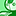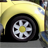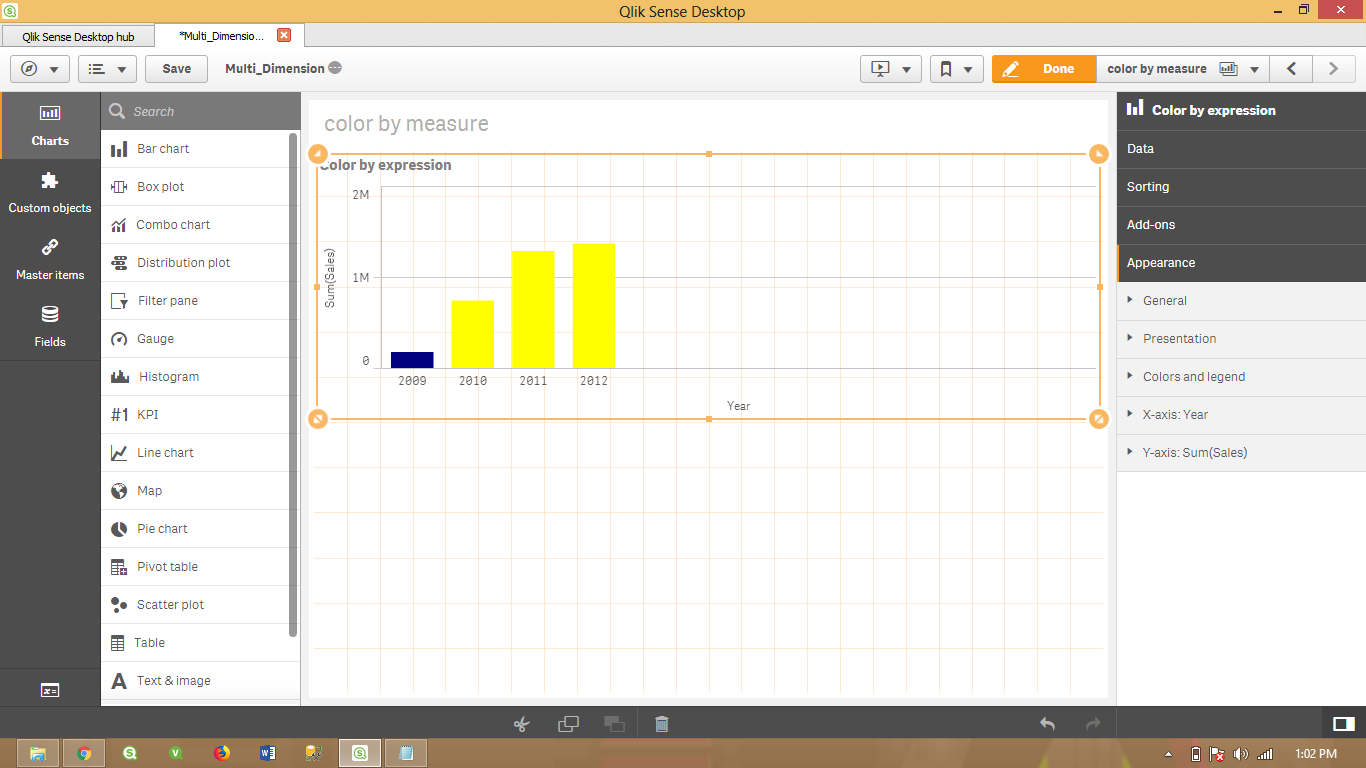# New to Qlik Sense

If you’re new to Qlik Sense, start with this Discussion Board and get up-to-speed quickly.

Announcements
Don't miss the upcoming Q&A with Qlik session on Qlik Application Automation on November 16th! REGISTER NOW
cancel
Showing results for
Did you mean:Creator

## By Expression color in qliksense.

i tried this below expression.

=if(Date(Date_1)>Today(),If((NetWorkDays(Date(Date_1),Today()))<10,'0-10',If((NetWorkDays(Date(Date_1),Today()))<20,'10-20','+20')),

If(((NetWorkDays(Date(Date_1),Today()-1))<10)*-1,'0-(10)',

If(((NetWorkDays(Date(Date_1),Today()-1))<20)*-1,'(10)-(20)','(20)+')))

we required + Values should be Green() and - Values be in Red.

11 RepliesSpecialist III

Hi Dilip,

=if you are using the same expression in Measure then you can try something like this in Color By Expression :

if(Column(1)>0,Green(),red())

if different expression in the Measure then can you check you're if condition.

=if(Date(Date_1)>Today() AND  If((NetWorkDays(Date(Date_1),Today()))<10,'0-10',

if(Date(Date_1)>Today() AND If((NetWorkDays(Date(Date_1),Today()))<20,'10-20','+20')),

If(((NetWorkDays(Date(Date_1),Today()-1))<10)*-1,'0-(10)',

If(((NetWorkDays(Date(Date_1),Today()-1))<20)*-1,'(10)-(20)','(20)+')))

Thanks,

MukramCreator
Author

Thank you for comment.

we tried but not expected resultsMVP

Which Version you are using probably if it is old , you are not able to get color by expression try to load latest version and check.

VikasSpecialist III

Hi,

can you attach sample file let me check the if condition

Thanks,

Mukram.Creator
Author

Qliksense 3.2 SR4Creator
Author

we can not attach the file . its confidentialCreator
Author

I'm using in Dimension.Creator III

How to chnage color in qlik sense by expression:-

Hi,

Here I have attached a simple example how to change the color of graph based on expression in qlik sense

In my example ,

Dimesion-----year(2009,2010,2011,2012)

Measure----Sales(Sum)

Go to:----

Appereances----colors and legands--------colors(select custom)---by expression-----

then apply whatever condition you want to apply in graph.

I have given simple example of expression:

if(Year='2009',Blue(),Yellow())Creator
Author

thank you vivek anand,

Im Using calculated Dimension as

=if(Date(Date_1)>Today() AND  If((NetWorkDays(Date(Date_1),Today()))<10,'0-10',

if(Date(Date_1)>Today() AND If((NetWorkDays(Date(Date_1),Today()))<20,'10-20','+20')),

If(((NetWorkDays(Date(Date_1),Today()-1))<10)*-1,'0-(10)',

If(((NetWorkDays(Date(Date_1),Today()-1))<20)*-1,'(10)-(20)','(20)+')))